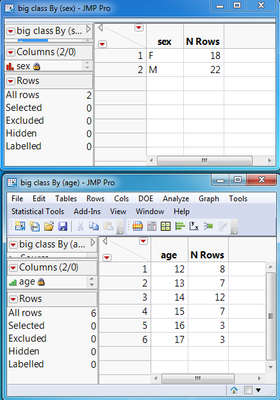Our World Statistics Day conversations have been a great reminder of how much statistics can inform our lives. Do you have an example of how statistics has made a difference in your life? Share your story with the Community!
Choose Language Hide Translation Bar

## Get a list of the unique values in a column, matrix, or list

Problem

Problem 1:  I need to get a list of the unique values in a data table column.

Problem 2:  I need to get a list of the unique values in a JSL data structure (list or matrix)

Solution

For problem 1:

Solution 1:  Use summary tables.  The JSL below shows how to use Summary() to get a table that will have a row with each unique value in a single column.   You can then do further JSL table commands to get those values from the summary table.

``````_dt = Open( "\$sample_data/big class.jmp" );
//create a summary table of the :sex column
_dt1 = _dt << Summary(
Group( :sex ),
Freq( "None" ),
Weight( "None" )
);
//create a summary table of the :age column
_dt2 = _dt << Summary(
Group( :age ),
Freq( "None" ),
Weight( "None" )
);``````

``

Problem 2:
Now,  instead of a data table you have a JMP list or matrix:
``````sexlist = {"F", "M", "M", "F", "F", "M", "M", "F", "M"};
agelist = [14, 11, 12, 13, 15, 17, 15, 11, 12];``````
the complicated ways to do this would be:
a) put the values in the list into a new data table column, and then use the summary approach, OR
b) write a somewhat complicated for loop to search for and record unique values

Fortunately, there is an easier way, using associative arrays.
``````Associative Array(sexlist) << Get Keys;
Associative Array(agelist) << Get Keys;``````
returns {"F", "M"} and {11, 12, 13, 14, 15, 17}, respectively (note that these are nice sorted list!).  If you would like the numeric values as a sorted vector, you can use
``Matrix(Associative Array(agelist) << Get Keys);``
which returns [11, 12, 13, 14, 15, 17].

This approach can also be used in place of Solution 1 for Problem 1:

Problem1, Solution 2:
``Associative Array(Column(_dt, "sex") << Get Values) << Get Keys;Matrix(Associative Array(Column(_dt, "age")<< Get Values)<<Get Keys);``
returns {"F", "M"} and [11, 12, 13, 14, 15, 17]., respectively. What this is doing is
• get the values from the specified column as a list (if the column is character) or a matrix (if the column is numeric)
• turn that list or martrix into an associative array
• get the keys for the associative array, which in this case are the unique levels of the values in the column, returning that as a list
• (if Matrix() is used on the resulting list, it will only work if the values in the list are all numbers).

Summarize() is way much more efficient than associative array, it is very obvious with 2 million rows of data (1sec vs 8sec time lapse). If performance is critical, use Summarize() whenever possible.

Summarize( dt, smrz1 = by( :sex ) ); Summarize( dt, smrz2 = by( :age ) );

* Summarize() does not create intermediate data table as << Summary

Associative Array is great in the way that it saves the effort to put the list/matrix into data table, this is perfect solution for small data size.

Article Labels

There are no labels assigned to this post.

Article Tags
Contributors